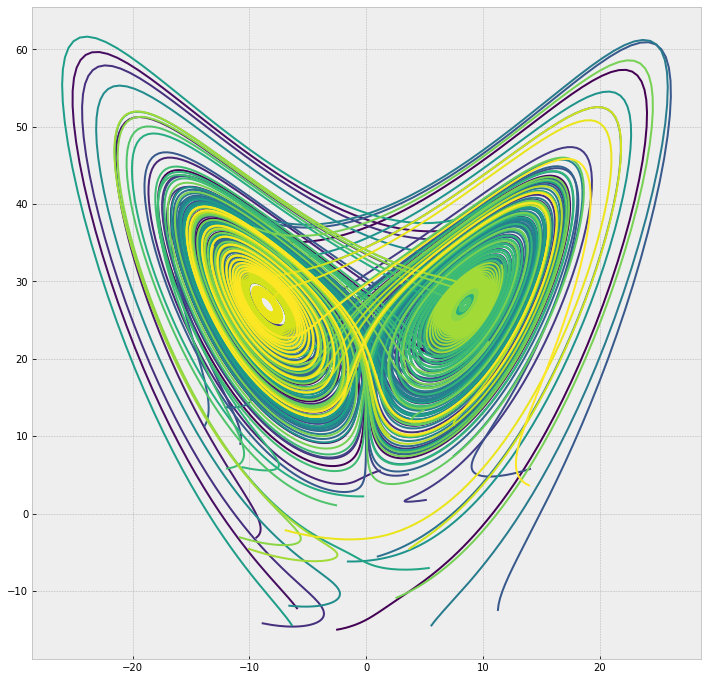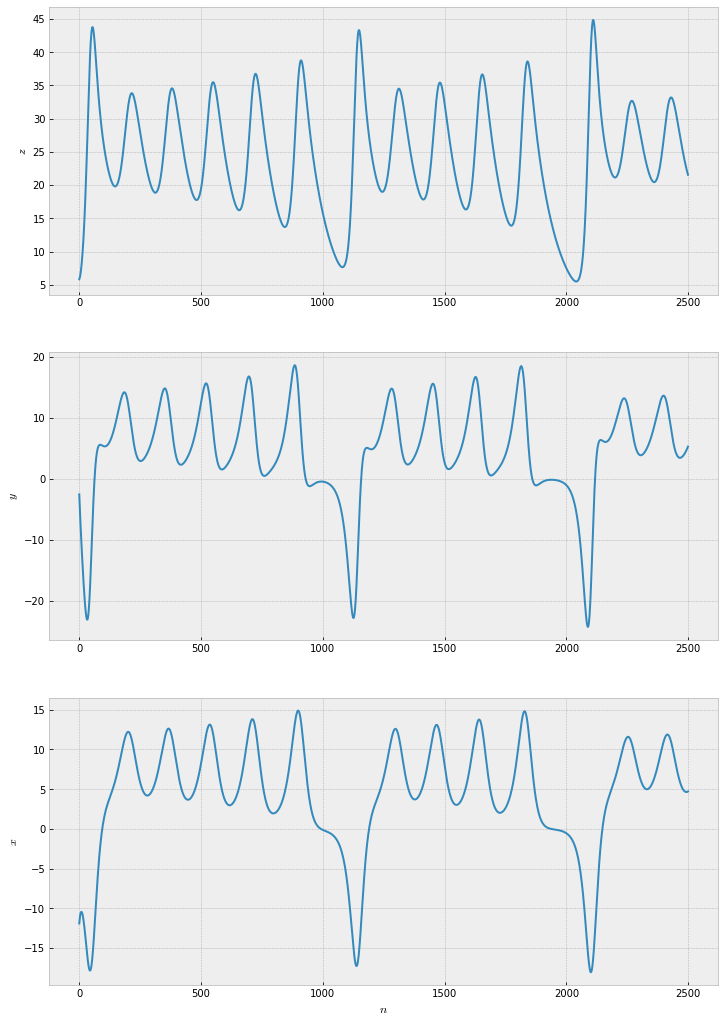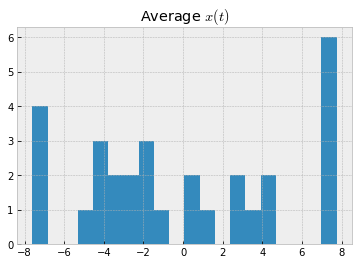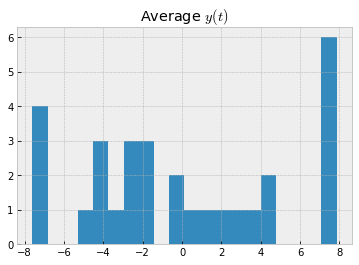# Lorenz Attractor ¶

We start with exploring Lorenz differential equations (also known as Lorenz attractor) using Python through examples, look at extremely complicated, aperiodic, non-transient and locally unstable trajectories created by Lorenz attractor.

Almost every book on chaos or non-linear dynamics contain the Lorenz differential equations, prints the unmistakable image of the attractor, or mentions the "butterfly effect". It has been intensely studied by scientist from many disciplines since its publication by Edward Lorenz in 1963. In it, he described a simplified mathematical model for atmospheric convection  . Nowadays, these equations are simply known as Lorenz attractor. However, for many years scientist have argued if Lorenz attractor was truly chaos or an artifact of exponential and explosive amplifications of numerical truncation errors. The existence of Lorenz attractor was finally settled by Tucker in 2002  .

Lorenz was a meteorologist and a mathematician in search of a model that was capable of generating long stretches of data statistically similar to atmospheric conditions. So, he set out to build a model with an aperiodic solution that was simple enough for the computing power available at the time. After a long search, Lorenz came up with the now-famous set of equations  :

\begin{aligned} \dot{x} & = \sigma(y-x) \\ \dot{y} & = \rho x - y - xz \\ \dot{z} & = -\beta z + xy \end{aligned}

where variables ($x$, $y$, $z$) and parameters ($\sigma$, $\rho$, $\beta$) are all real numbers.

Like many nonlinear equations, there isn't an analytical solution for Lorenz attractor. So, with practicality in mind, I will use numerical methods (more specifically discrete numerical methods with finite time-steps) to solve these equations and share some of the surprising features using the generated time-series. Besides, time-series are often the only observable data available to quantify the complicated dynamics in real life situations or experiments. So, let's jump right into it!

In :
import utils

N=30
t, x_t = utils.solve_lorenz(10.0, 8.0/3, 28.0, N)


Above, we computed (numerically solved) 30 trajectories with random initial conditions for $x$, $y$, $z$ using $\sigma = 10$, $\rho = 2.\overline{6}$, and $\beta = 28$. I will not get into what variables ($x$, $y$, $z$) and parameters ($\sigma$, $\rho$, $\beta$) represent. However, for wide range of values of parameters numerical solutions of the equations above generate extremely complicated trajectories. Let's plot them:

In :
%matplotlib inline
import numpy as np
import matplotlib.pyplot as plt

colors = plt.cm.viridis(np.linspace(0, 1, N))
plt.figure(figsize=(12, 12))

plt.style.use('bmh')

for i in range(N):
x, y, z = x_t[i,:,:].T
plt.plot(x, z, '-', c=colors[i])This is the famous and unmistakable butterfly figure plotted in two dimensions. Notice that each trajectory exhibits aperiodic behavior, not cross itself if we consider the full three-dimensional picture.

In :
plt.style.use('bmh')


Matplotlib is a powerful plotting library which produces publication quality figures. It can be very complex and time-consuming to create aesthetically pleasing plots. Luckily, we can take shortcuts and use ready-made styles. One particular style is called "bmh" which offer many improvements over the default settings of matplotlib and works well with Jupyter notebooks. This style comes from Bayesian Methods for Hackers  book. I find it particularly suiting for scientific graphing and I will be using it frequently.

The aperiodic behavior is more apparent when we plot each variable separately.

In :
plt.figure(figsize=(12, 18))
x, y, z = x_t[20,:,:].T

ax1 = plt.subplot(311)
ax1.plot(z)
ax1.set_ylabel('$z$')

ax2 = plt.subplot(312, sharex=ax1)
ax2.plot(y)
ax2.set_ylabel('$y$')

ax3 = plt.subplot(313, sharex=ax1)
ax3.plot(x)
ax3.set_ylabel('$x$')
ax3.set_xlabel('$n$')

plt.show()The plots above show the variables variables ($x$, $y$, $z$) of a turbulent trajectory in three dimensional space with the following properties:

1. The trajectories are not periodic . One can integrate over long periods of time and the trajectory would not cross itself in three dimensional space.
2. The trajectories are not transient , meaning they do not settle into periodic or stationary behavior.
3. The behavior of the trajectories does not depend on our choice of initial conditions. In other words, the system is locally unstable .
4. Most importantly, small changes in initial conditions lead to great difference in long-term called the butterfly effect .

For wide ranges of values of parameters ($\sigma$, $\rho$, $\beta$), the properties above hold true.

Creating histograms of the average positions (across different trajectories) show that, on average, the trajectories swirl about the attractors.

In :
xyz_avg = x_t.mean(axis=1)
# xyz_avg.shape

plt.hist(xyz_avg[:,0], 20)
plt.title('Average $x(t)$');In :
plt.hist(xyz_avg[:,1], 20)
plt.title('Average $y(t)$');###### Summary
We start with exploring Lorenz differential equations (also known as Lorenz attractor) using Python through examples, look at extremely complicated, aperiodic, non-transient and locally unstable trajectories created by Lorenz attractor.
March 29, 2019
###### Recent Posts
• Lorenz Attractor We start with exploring Lorenz differential equations (also known as Lorenz attractor) using...
• Poincaré Surface of Section Poincaré surface of section (also referred to as Poincaré section or Poincaré map) is powerful...
• Delay Embedding We try to reconstruct a chaotic dynamical system from a time-series using Taken's delay...